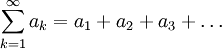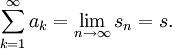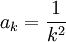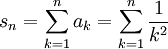# Convergence of Infinite Series

Previous: Introduction to Infinite Series

Next: The Geometric Series

Our first task is to establish, given an infinite series, whether or not it converges. Knowing whether or not a given infinite series converges requires a definition of convergence.

Definition: Convergence of an Infinite Series
Suppose we are given an infinite seriesLet sn denote thepartial sum of the infinite series:If the sequenceis convergent andexists, then the infinite seriesis convergent andMoreover, the number s, if it exists, is referred to as the sum of the series. A series that is not convergent is referred to as divergent.

The above definition could be made more precise with a more careful definition of a limit, but this would go beyond the scope of what we need. But our definition provides us with a method for testing whether a given infinite series converges: suppose we can construct an expression,, for the partial sum of a given infinite series, and we want to know if the series converges. If the limittends to a finite number, the series converges. Otherwise, the series diverges.

## Example: Partial Sums of 1/k2

Unfortunately, it is often difficult to find a formula forthat we can use for this test. But to give us a sense of whether a given series converges, we can use a computer to plotfor a specified number of terms in the sequence. For example, let's considerand use a computer to try to guess what the partial sumconverges to when we lettend to infinity. The following widget calculates the partial sums for us, so we can use it do this. The widget plots the partial sums of 1 / k² from 1 to n. Choose a large value of n and try to make a guess as to whether or not the series converges.

You should be able to, using the widget above, guess whether the series converges, and if it does, what the series converges to by looking at what the partial sums converge to when n gets large (try using "infinity": the series converges toover 6). However, simply plotting the partial sums, although insightful, does not give us an answer to our first key question: given an infinite series, does it converge? To do this, we could find an expression for sn and take a limit. We will demonstrate this approach in the following section on the geometric series.

Previous: Introduction to Infinite Series

Next: The Geometric Series